Related Articles

# Draw Composition of Plots Using the patchwork Package in R

• Last Updated : 28 Jul, 2021

In this article, we will discuss how to draw a composition of plots using the patchwork package in R programming language. The patchwork package makes it easier to plot different ggplots in a single graph. We will require both ggplot2 packages for plotting our data and the patchwork package to combine the different ggplots.

Let us first draw all the plots normally and independently.

Example: Plotting the dataset in a bar plot

## R

 `library``(ggplot2)``library``(patchwork)`` ` `data``(iris)`` ` `head``(iris)`` ` `# Plotting the bar chart``gfg1 <- ``ggplot``(iris, ``aes``(Sepal.Length, Petal.Length, color = Species)) + ``geom_bar``(stat = ``"identity"``)`` ` `gfg1`

Output :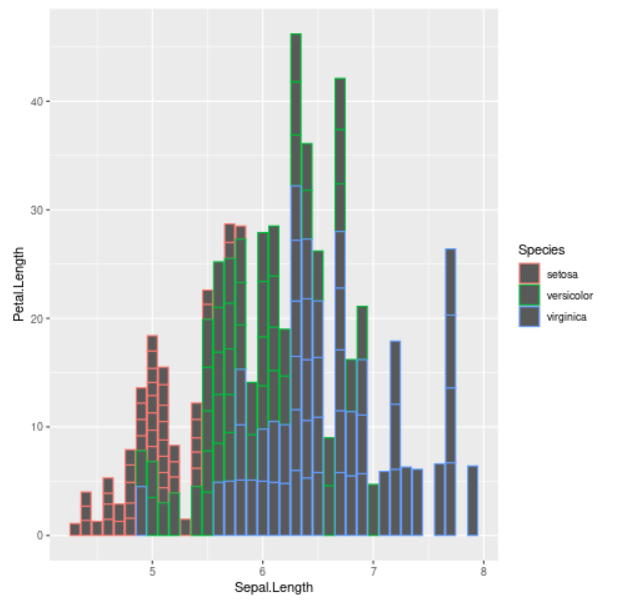Bar plot

Example: Plotting the dataset in a scatterplot

## R

 `library``(ggplot2)``library``(patchwork)`` ` `data``(iris)`` ` `head``(iris)`` ` `# Scatterplot``gfg2 <- ``ggplot``(iris, ``aes``(Sepal.Length, Petal.Length, ``                         ``color = Species)) + ``geom_point``()`` ` `gfg2`

Output :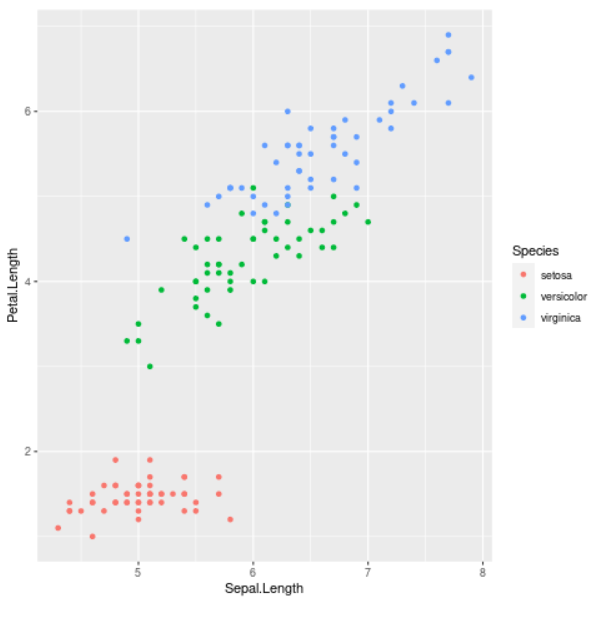Scatterplot

Example: Plotting the dataset in a line plot

## R

 `library``(ggplot2)``library``(patchwork)`` ` `data``(iris)`` ` `head``(iris)`` ` `# Line plot``gfg3 <- ``ggplot``(iris, ``aes``(Sepal.Length, Petal.Length, ``                         ``color = Species)) + ``geom_line``()`` ` `gfg3`

Output :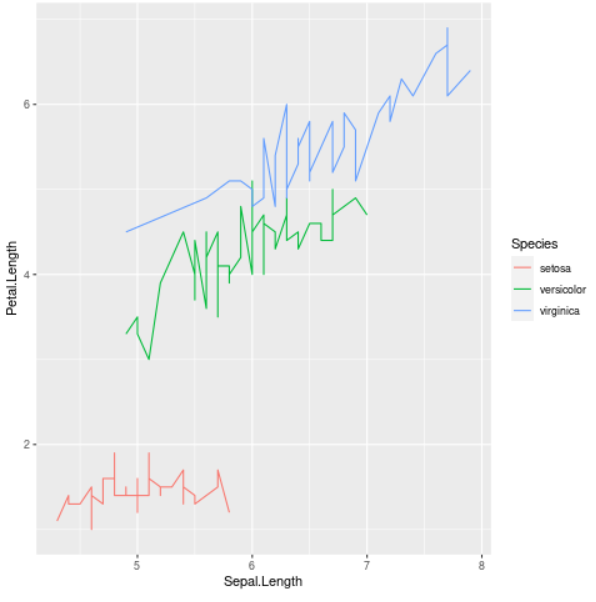Line plot

Now let us look at how these three plots can be combined in various orientations.

## Plotting Vertically

The vertical orientation combines the plots and these plots are placed side by side with each other. We will take the above ggplot2 plots and add them to arrange them vertically. The + operator is used to arrange these plots side by side in the same graph.

Example: Vertically arrange plots in the same frame

## R

 `library``(ggplot2)``library``(patchwork)`` ` `data``(iris)`` ` `head``(iris)`` ` `gfg1 <- ``ggplot``(iris, ``aes``(Sepal.Length, Petal.Length, ``                         ``color = Species)) + ``geom_bar``(stat = ``"identity"``)`` ` `gfg2 <- ``ggplot``(iris, ``aes``(Sepal.Length, Petal.Length, ``                         ``color = Species)) + ``geom_point``()`` ` `gfg3 <- ``ggplot``(iris, ``aes``(Sepal.Length, Petal.Length, ``                         ``color = Species)) + ``geom_line``()`` ` `# Combination of plots by arranging them side by side``gfg_comb_1 <- gfg1 + gfg2 + gfg3`` ` `gfg_comb_1`

Output :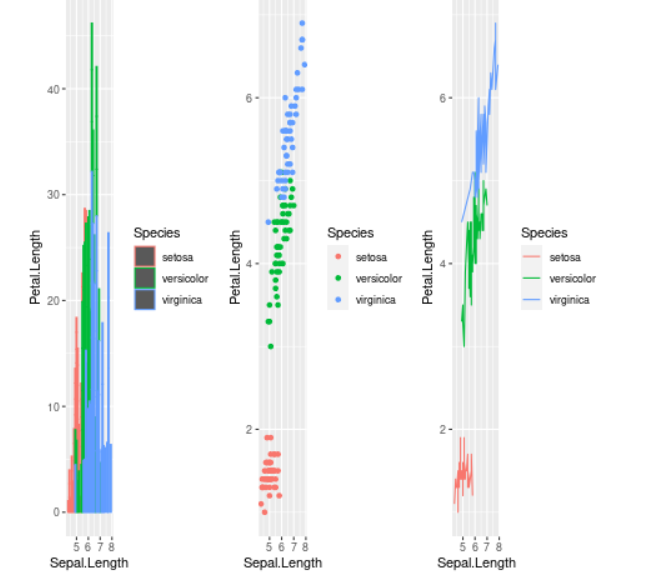Vertical orientation

## Arranging with different widths

The second combination of these plots would be arranging them in different widths in multiple rows and columns in the same graph window. The different width orientation arranges the plots in different lines according to widths. Basically, the first plot is placed on the first line and the other two plots are placed on the next line horizontally.

We use a slash(/) operator at that position where we want to start a new line. So we placed it after the first plot. Then, we use add(+) operator to arrange the other two plots horizontally on the next new line.

Example: Arranging plots with different widths

## R

 `library``(ggplot2)``library``(patchwork)`` ` `data``(iris)`` ` `head``(iris)`` ` `gfg1 <- ``ggplot``(iris, ``aes``(Sepal.Length, Petal.Length,``                         ``color = Species)) + ``geom_bar``(stat = ``"identity"``)`` ` `gfg2 <- ``ggplot``(iris, ``aes``(Sepal.Length, Petal.Length, ``                         ``color = Species)) +``geom_point``()`` ` `gfg3 <- ``ggplot``(iris, ``aes``(Sepal.Length, Petal.Length, ``                         ``color = Species)) +``geom_line``()`` ` `# Arranging the plots in different widths``gfg_comb_2 <- gfg1 / (gfg2 + gfg3)`` ` `gfg_comb_2`

Output :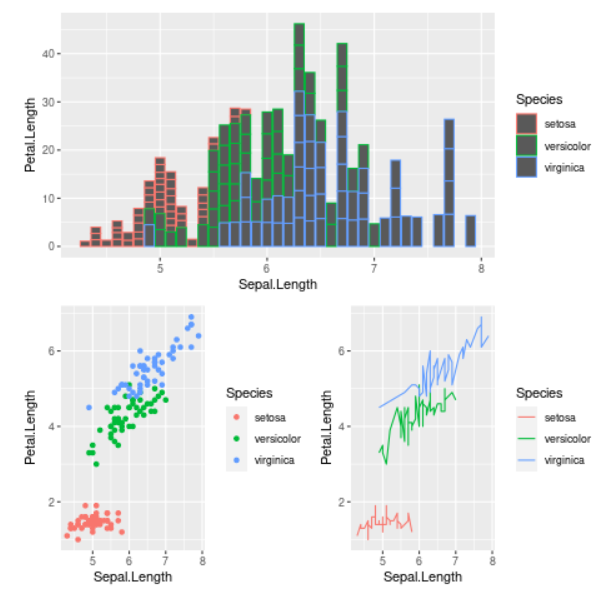Different widths orientation

## Arranging with different heights

The third combination of these plots would be arranging them in different heights in multiple rows and columns in the same graph window. The different height orientation arranges the plots in different lines according to heights. Basically, the first two plots are arranged horizontally and the last plot here is placed below the second plot.

We use the add(+) operator to arrange the first two plots horizontally. Then we use the slash (/) operator for the third plot to place it on a new line below the second plot.

Example: Arranging plots with different heights

## R

 `library``(ggplot2)``library``(patchwork)`` ` `data``(iris)`` ` `head``(iris)`` ` `gfg1 <- ``ggplot``(iris, ``aes``(Sepal.Length, Petal.Length,``                         ``color = Species)) + ``geom_bar``(stat = ``"identity"``)`` ` `gfg2 <- ``ggplot``(iris, ``aes``(Sepal.Length, Petal.Length,``                         ``color = Species)) +``geom_point``()`` ` `gfg3 <- ``ggplot``(iris, ``aes``(Sepal.Length, Petal.Length,``                         ``color = Species)) + ``geom_line``()`` ` `# Arrangement of plots according to ``# different heights``gfg_comb_3 <- gfg1 + gfg2 / gfg3`` ` `gfg_comb_3`

Output :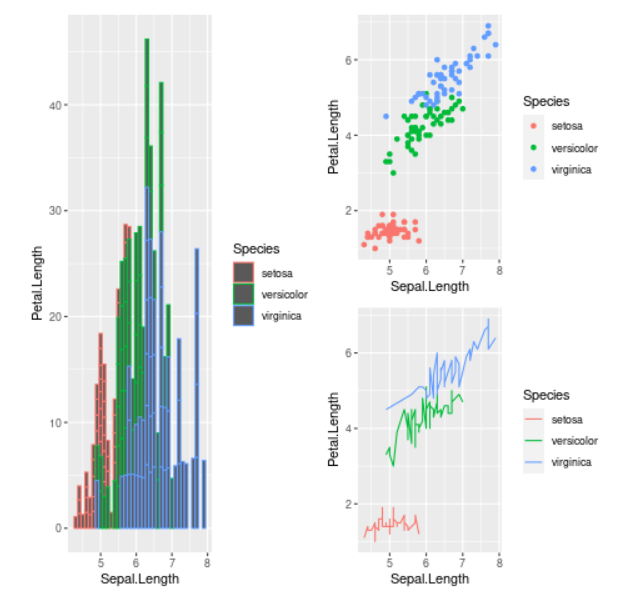Different heights orientation

## Plotting Horizontally

The last combination of plots is to arrange them horizontally. These plots are placed one below the other. We will use the slash(/) operator to place these plots in a new line, one below the other plot.

Example: Arranging plots vertically

## R

 `library``(ggplot2)``library``(patchwork)`` ` `data``(iris)`` ` `head``(iris)`` ` `gfg1 <- ``ggplot``(iris, ``aes``(Sepal.Length, Petal.Length, color = Species)) + ``geom_bar``(stat = ``"identity"``)`` ` `gfg2 <- ``ggplot``(iris, ``aes``(Sepal.Length, Petal.Length, color = Species)) + ``geom_point``()`` ` `gfg3 <- ``ggplot``(iris, ``aes``(Sepal.Length, Petal.Length, color = Species)) + ``geom_line``()`` ` `# Combination of plots by arranging them ``# horizontally``gfg_comb_4 <- gfg1 / gfg2 / gfg3`` ` `gfg_comb_4`

Output :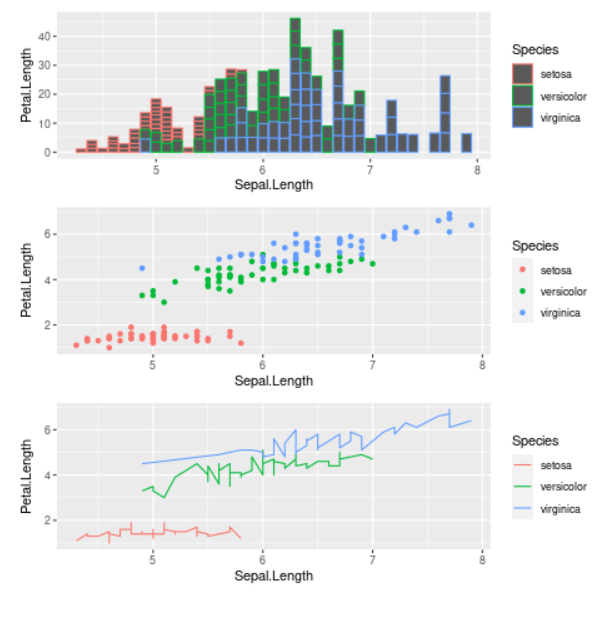Horizontal orientation

My Personal Notes arrow_drop_up